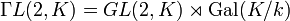# General semilinear group of degree two

(Redirected from GammaL(2,q))
Let$K$ be a field. The general semilinear group of degree two over$K$, denoted$\Gamma L(2,K)$, is defined as the general semilinear group of degree two over$K$. Explicitly, it is the external semidirect product:$\Gamma L (2,K) = GL(2,K) \rtimes \operatorname{Aut}(K)$
where$GL(2,K)$ denotes the general linear group of degree two and$\operatorname{Aut}(K)$ is the group of field automorphisms of$K$ acting entry-wise on the matrices.
If$k$ is the prime subfield of$K$, and$K$ is a Galois extension of$k$ (note that this case always occurs for$K$ a finite field), then$\operatorname{Aut}(K) = \operatorname{Gal}(K/k)$ (the Galois group) and we get:$\Gamma L (2,K) = GL(2,K) \rtimes \operatorname{Gal}(K/k)$